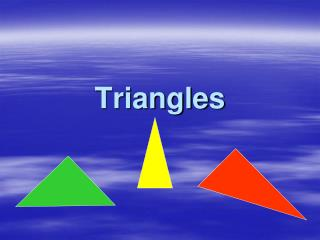DownloadDownload PresentationTriangles

# Triangles

Télécharger la présentation## Triangles

- - - - - - - - - - - - - - - - - - - - - - - - - - - E N D - - - - - - - - - - - - - - - - - - - - - - - - - - -
##### Presentation Transcript

1. Triangles

2. Take a rectangular piece of paper.

3. Take a rectangular piece of paper. Fold it in half

4. Take a rectangular piece of paper. Fold it in half Use a ruler to draw a line from the top of the fold to the bottom right hand corner.

5. Take a rectangular piece of paper. Fold it in half Use a ruler to draw a line from the top of the fold to the bottom right hand corner. Cut along the line.

6. You should now have 3 triangles

7. Can you prove that this is a line of symmetry?

8. Does this triangle have any other line of symmetry?

9. No it doesn’t. It only has the one.

10. How can we prove that line ac is equal to line bc? By folding along the line of symmetry. If you haven’t tried it, do it now. d b a c

11. d b a b This type of triangle, where two sides are equal, has a special name. It is called an ISOSCELES triangle c

12. d b a b Stick this triangle into your jotter and name it ISOSCELES triangle. Now use the other two pieces of the rectangle to make another isosceles triangle. c

13. Your new triangle should look like this. How do we know it is isoscles?

14. Which of these triangles do you think is isosceles?

15. These are the isosceles triangles. Write in your jotter how we can describe an isosceles triangle and how we can prove it is isosceles.